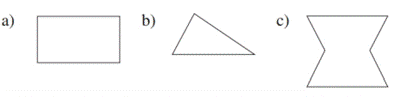Chapter 2.6, Problem 5EElementary Geometry For College St...

7th Edition
Alexander + 2 others
ISBN: 9781337614085

Solutions

Chapter
SectionElementary Geometry For College St...

7th Edition
Alexander + 2 others
ISBN: 9781337614085
Textbook Problem

Which geometric figures have symmetry with respect to atleast one line?To determine

To choose:

The geometric figures having symmetry with respect to at least one line.

Explanation

Consider the geometric figure (a).

Figure (1)

From figure (1), the geometric figure (a) has symmetry with respect to line l and line m.

Consider the geometric figure (c).

Still sussing out bartleby?

Check out a sample textbook solution.

See a sample solution

The Solution to Your Study Problems

Bartleby provides explanations to thousands of textbook problems written by our experts, many with advanced degrees!

Get Started

Differentiate. F(t)=AtBt2+Ct3

Single Variable Calculus: Early Transcendentals, Volume I

In Exercises 63-68, rationalize the denominator. 68. 2xy

Applied Calculus for the Managerial, Life, and Social Sciences: A Brief Approach

Prove the identity. 55. sin1cos=csc+cot

Single Variable Calculus: Early Transcendentals

True or False: is monotonic.

Study Guide for Stewart's Multivariable Calculus, 8th

True or False: If f′(x) = g′(x), then f(x) = g(x).

Study Guide for Stewart's Single Variable Calculus: Early Transcendentals, 8th